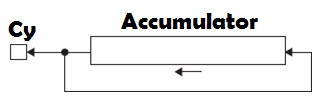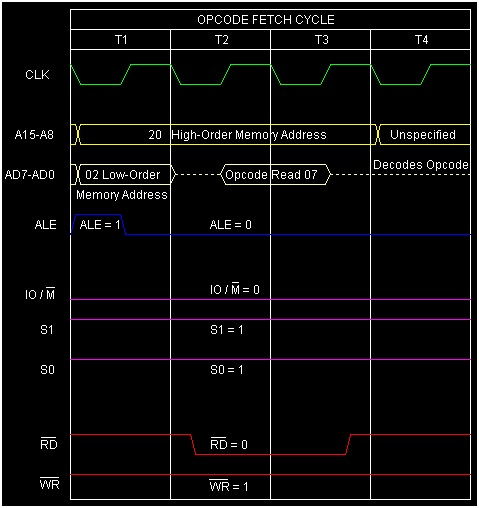# Instruction type RLC in 8085 Microprocessor

In 8085 Instruction set, there is one mnemonic RLC stands for “Rotate Left Accumulator”. It rotates the Accumulator contents to the left by 1-bit position. The following Fig. shows the operation explicitly.In this fig. it has been depicted that the most significant bit of the Accumulator will come out and left rotate will create an empty space at the least significant bit place and this come out bit will be copied at the empty bit place and also on the Cy bit in the flag register. Thus, Cy flag gets a copy of the bit moved out from the MS bit position. Notice that Cy flag is not involved in the rotation, and it is only 8-bit rotation of Accumulator contents. Only Cy flag is affected by this instruction execution.

Mnemonics, Operand Opcode(in HEX) Bytes
RLC 07 1

This instruction can be used in following different case studies.

• To check whether the number is positive or negative. As the most significant of the Accumulator content holds the sign bit.

• To perform multiplication by 2, rotate the Accumulator to left. It works correctly for unsigned numbers, as long as the MS bit of Accumulator is a 0 before rotation. As we know that multiplication by 2n results n-bit left shift of the number.

Let us discuss some examples on this mnemonic usage.

## Example 1

35H --->    0011 0101
0 0110 1010 ---> 6AH


Before After

(A)

35H 6AH

(Cy)

Any value 0

2002 07 RLC Rotate Left Accumulator

Here the accumulation content has been doubled as we had 1-bit left shift and the MSB was 0.

## Example 2

95H --->    1001 0101
1 0010 1011 ---> 2BH


Before After

(A)

95H 2BH

(Cy)

Any value 1

Here, in this example, the Accumulator value has not been doubled, as most significant bit of theAccumulator was a 1 before the rotation.